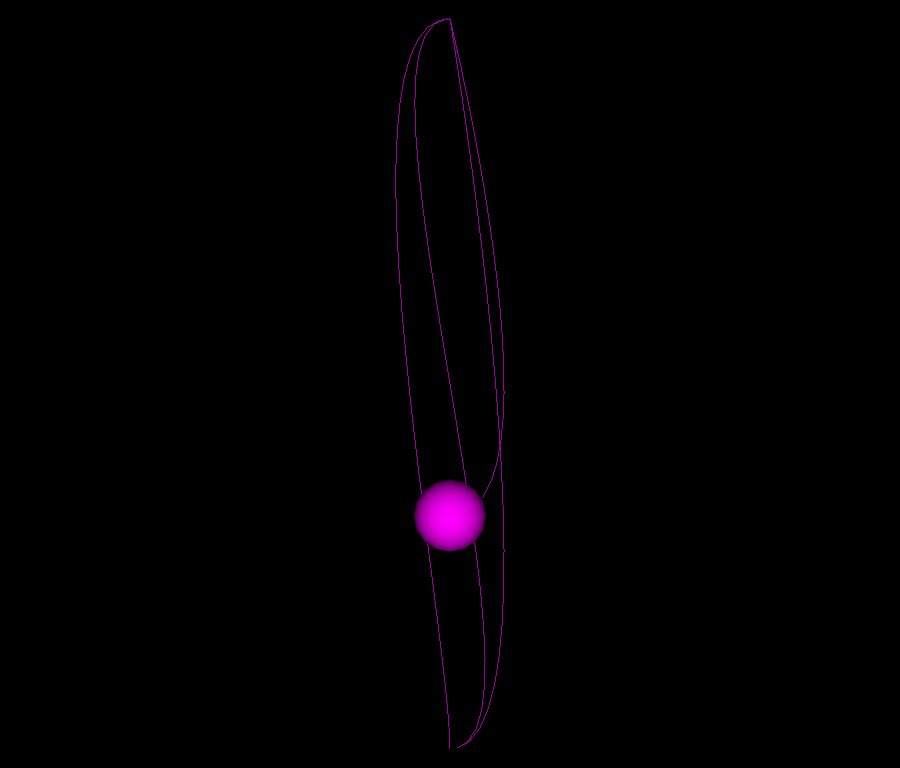# Making a custom interpolator#

Keyframe animation using custom interpolator.

```import numpy as np

from fury import actor, window
from fury.animation import Animation, helpers
```

## Implementing a custom interpolator#

A keyframe interpolator function must return a function which take the time as an argument and returns a value. It’s recommended to import fury.animation.helpers, which has some useful functions that would help to implement the interpolator.

In glTF, animations using cubic spline interpolator needs at least two points, and each point has two tangent vectors. The interpolation equation for such data is in the glTF tutorials below: https://github.khronos.org/glTF-Tutorials/gltfTutorial/gltfTutorial_007_Animations.html#cubic-spline-interpolation

Tangent based cubic spline interpolation function:

```>>> def cubicSpline(previousPoint, previousTangent, nextPoint, nextTangent,
>>>                   interpolationValue):
>>>     t = interpolationValue
>>>     t2 = t * t
>>>     t3 = t2 * t
>>>     return (2 * t3 - 3 * t2 + 1) * previousPoint +
>>>            (t3 - 2 * t2 + t) * previousTangent +
>>>            (-2 * t3 + 3 * t2) * nextPoint +
>>>            (t3 - t2) * nextTangent
```

First we create a function that must take a dict object that contains the animation keyframes when initialized as follows:

```>>> def tan_cubic_spline_interpolator(keyframes):
>>>     ...
>>>     def interpolate(t):
>>>           return interpolated_value
>>>     return interpolator
```

Note: Also any other additional arguments are ok, see spline_interpolator Second step is to implement the interpolate closure that only takes the current time as input.

```def tan_cubic_spline_interpolator(keyframes):
# First we must get ordered timestamps array:
timestamps = helpers.get_timestamps_from_keyframes(keyframes)

# keyframes should be on the following form:
# {
# 1: {'value': ndarray, 'in_tangent': ndarray, 'out_tangent': ndarray},
# 2: {'value': np.array([1, 2, 3], 'in_tangent': ndarray},
# }
# See here, we might get incomplete data (out_tangent) in the second
# keyframe. In this case we need to have a default behaviour dealing
# with these missing data.
# Setting the tangent to a zero vector in this case is the best choice
for time in keyframes:
data = keyframes.get(time)
value = data.get('value')
if data.get('in_tangent') is None:
data['in_tangent'] = np.zeros_like(value)
if data.get('in_tangent') is None:
data['in_tangent'] = np.zeros_like(value)

def interpolate(t):
# `get_previous_timestamp`and `get_next_timestamp` functions take
# timestamps array and current time as inputs and returns the
# surrounding timestamps.
t0 = helpers.get_previous_timestamp(timestamps, t)
t1 = helpers.get_next_timestamp(timestamps, t)

# `get_time_tau` function takes current time and surrounding
# timestamps and returns a value from 0 to 1
dt = helpers.get_time_tau(t, t0, t1)

time_delta = t1 - t0

# to get a keyframe data at a specific timestamp, use
# `keyframes.get(t0)`. This keyframe data contains `value` and any
# other data set as a custom argument using keyframe setters.
# for example:
# >>> animation = Animation()
# >>> animation.set_position(0, np.array([1, 1, 1]),
# >>>                       custom_field=np.array([2, 3, 1]))
# In this case `keyframes.get(0)` would return:
# {'value': array(1, 1, 1), 'custom_field': array(2, 3, 1)}
#
# now we continue with the cubic spline equation.
p0 = keyframes.get(t0).get('value')
tan_0 = keyframes.get(t0).get('out_tangent') * time_delta
p1 = keyframes.get(t1).get('value')
tan_1 = keyframes.get(t1).get('in_tangent') * time_delta
# cubic spline equation using tangents
t2 = dt * dt
t3 = t2 * dt
return (
(2 * t3 - 3 * t2 + 1) * p0
+ (t3 - 2 * t2 + dt) * tan_0
+ (-2 * t3 + 3 * t2) * p1
+ (t3 - t2) * tan_1
)

return interpolate

scene = window.Scene()
showm = window.ShowManager(
scene, size=(900, 768), reset_camera=False, order_transparent=True
)
```

## Cubic spline keyframes data same as the one you get from glTF file.#

```#               t    in tangent     position                   out tangent
translation = [
[0.0, [0.0, 0.0, 0.0], [3.3051798, 6.640117, 0.0], [1.0, 0.0, 0.0]],
[1.0, [0.0, 0.0, 0.0], [3.3051798, 8.0, 0.0], [-1.0, 0.0, 0.0]],
[2.0, [-1.0, 0.0, 0.0], [3.3051798, 6.0, 0.0], [1.0, 0.0, 0.0]],
[3.0, [0.0, 0.0, 0.0], [3.3051798, 8.0, 0.0], [-1.0, 0.0, 0.0]],
[4.0, [0, -1.0, 0.0], [3.3051798, 6.0, 0.0], [0.0, 0.0, 0.0]],
]
```

Initializing an `Animation` and adding sphere actor to it.

```animation = Animation(motion_path_res=100)

sphere = actor.sphere(np.array([[0, 0, 0]]), (1, 0, 1), radii=0.1)

```

## Setting position keyframes#

```for keyframe_data in translation:
t, in_tan, pos, out_tan = keyframe_data
# Since we used the name 'in_tangent' and 'out_tangent' in the interpolator
# We must use the same name as an argument to set it in the keyframe data.
animation.set_position(t, pos, in_tangent=in_tan, out_tangent=out_tan)
```

Set the new interpolator to interpolate position keyframes

```animation.set_position_interpolator(tan_cubic_spline_interpolator)
```

adding the animation to the show manager.

```showm.add_animation(animation)

interactive = False

if interactive:
showm.start()

window.record(scene, out_path='viz_keyframe_custom_interpolator.png', size=(900, 768))
```Total running time of the script: ( 0 minutes 0.114 seconds)

Gallery generated by Sphinx-Gallery